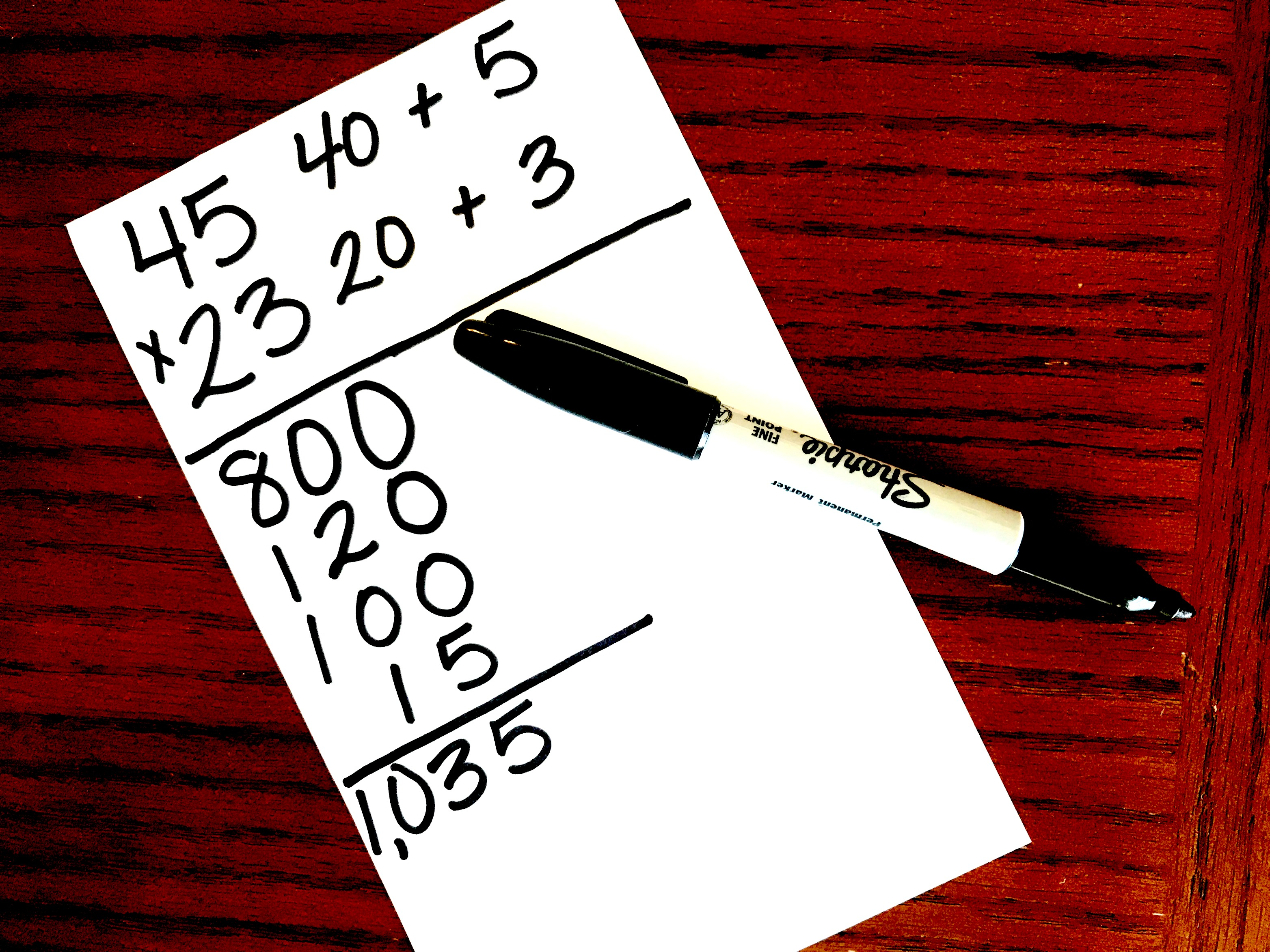Home » Math » Four Ways To Teach Multiplication with Free A Printable – Part 1 Partial Products

Four Ways To Teach Multiplication with Free A Printable – Part 1 Partial Products

The free printable can be found at the END of this post. Click on the blue “get your free printable here” button.

I’ve heard it from parents. I’ve even heard it from my husband the other day.

What happened to the old way of doing things?

We homeschool! And when it was time to teach my son to subtract with regrouping I taught him two different ways. And guess what?He doesn’t prefer the traditional way. He would rather subtract using partial difference, which is using place value to subtract.

It makes sense to him. It works for him. So that is how he will do it.

And when it comes time to teach him multiplication, I will be teaching him three different methods. Again, he will be able to choose what works best for him. And whatever he chooses, that will be what we will focus on.

Why Teach Different Methods?

We all think differently!

We approach problems in different ways.

Need a solution, your students’ can come up with 100 different ways to help!

So if there are numerous way to get to a math answer, why don’t we teach a few of them? See which one appeals the most to our kiddos way of thinking….and then let them run with it.

As long as they are consistently successful in a timely manner, why should it matter if they solving in a traditional way of the “new” way???

So what are some “new” ways you can teach multiplication??

I’m so glad you asked. Today is part one, of a four part series. We will cover partial products, area model, lattice multiplication, and last but not least…the traditional way. Each post will contain step by step directions you can print off, as well as activities that you will help your child practice this concept.

So, if you are looking for another way to teach multiplication, check out these other methods and interactive notebooks.

Download your Step by Step Instructions on How to Teach Area Model

How to Teach Multiplication Using Arrays and Base 10 Blocks

And the kids favorite, How to Multiply Using the Lattice Method

So let’s get started!!

Partial Products

I love using partial sums, partial differences, partial products, and even partial quotients strategies for doing math in my head. It is basically using place value to solve problems.

So how does it work with multiplication?

Step 1:

Take both numbers you will be multiplying and write them out in expanded form.• For the number 45 x 23, you would write out 40 + 5 and then 20 + 3.

Step 2:

Now, we use the numbers written in expanded form to multiply. Starting with the first number in the expanded form expression, you multiply it by both values of the

Start with the first number in the first expanded form expression, and then multiply it by both of the values in the second expanded form expression.• For 45 x 23, you would multiply 40 x 20 and write that answer underneath the main problem.• Then you would multiply 40 x 3, and write that answer underneath the previous answer.

Step 3:

Next, we move on to the second value in our first expanded form expression. We use this number and multiply it by both numbers in the second expanded form expression.• For 45 x 23, you would multiply  5 x 20 and write that answer underneath the previous answers.
• Then you would multiply 5 x 3, and write that answer underneath the previous answers.

*For this problem we are almost done, but if there are more values in the first expanded form expression, then you would repeat these steps until every value had been multiplied by the values in the second expression.

Step 4:

Finally, add up all the answers and you have your product.The name may sound scary, but it’s not too bad. Don’t forget to grab your free step by step instructions, and join us in exploring how to use area models to teach multiplication.

You’ve Got This Math

Rachel